# A 1.00 × 10² g aluminum block at 100.0℃ is placed in 1.00 × 10² g of water at 10.0℃

4. A 1.00 × 10² g aluminum block at 100.0℃ is placed in 1.00 × 10² g of water at 10.0℃. The final temperature of the mixture is 26.0℃. What is the specific heat of the aluminum?

5. Three metal fishing weights, each with a mass of 1.00 × 10² g and at a temperature of 100.0℃, are placed in 1.00 × 10² g of water at 35.0℃. The final temperature of the mixture is 45.0℃. What is the specific heat of the metal in the weights?

SOLUTION :

4.

Heat flows from higher temperature object to lower temperature object when they come

In contact. So, heat is transferred from aluminium at 100ºC to water at 10ºC.

As per energy system,

Heat given by aluminium = Heat taken by water.

=> ma * sa * (ti - tf) = mw * sw * (ff - ti

=> 1.00*10^2 * sa * (100 - 26) = 1*10^2 * 1.00 * (26 - 10)

=> 74.00*10^2 sa = 16.00*10^2

=> sa = 16.00/74.00 = 0.2162 cal/(g*ºC)

So, specific heat of aluminium is 0.2162 cal/g ºC (ANSWER)

5.

Heat flows from higher temperature object to lower temperature object when they come

In contact. So, heat is transferred from metal fishing weight at 100ºC to water at 35ºC.

As per energy system,

Heat given by metal fishing weight = Heat taken by water.

=> mm * sm * (ti - tf) = mw * sw * (tf - ti

=> 1.00*10^2 * sm * (100 - 45) = 1*10^2 * 1.00 * (45 - 35)

=> 55.00*10^2 sm = 10.00*10^2

=> sm = 10.00/55.00 = 0.1818 cal/(g*ºC)

So, specific heat of metal fishing weight is 0.1818 cal/g ºC (ANSWER)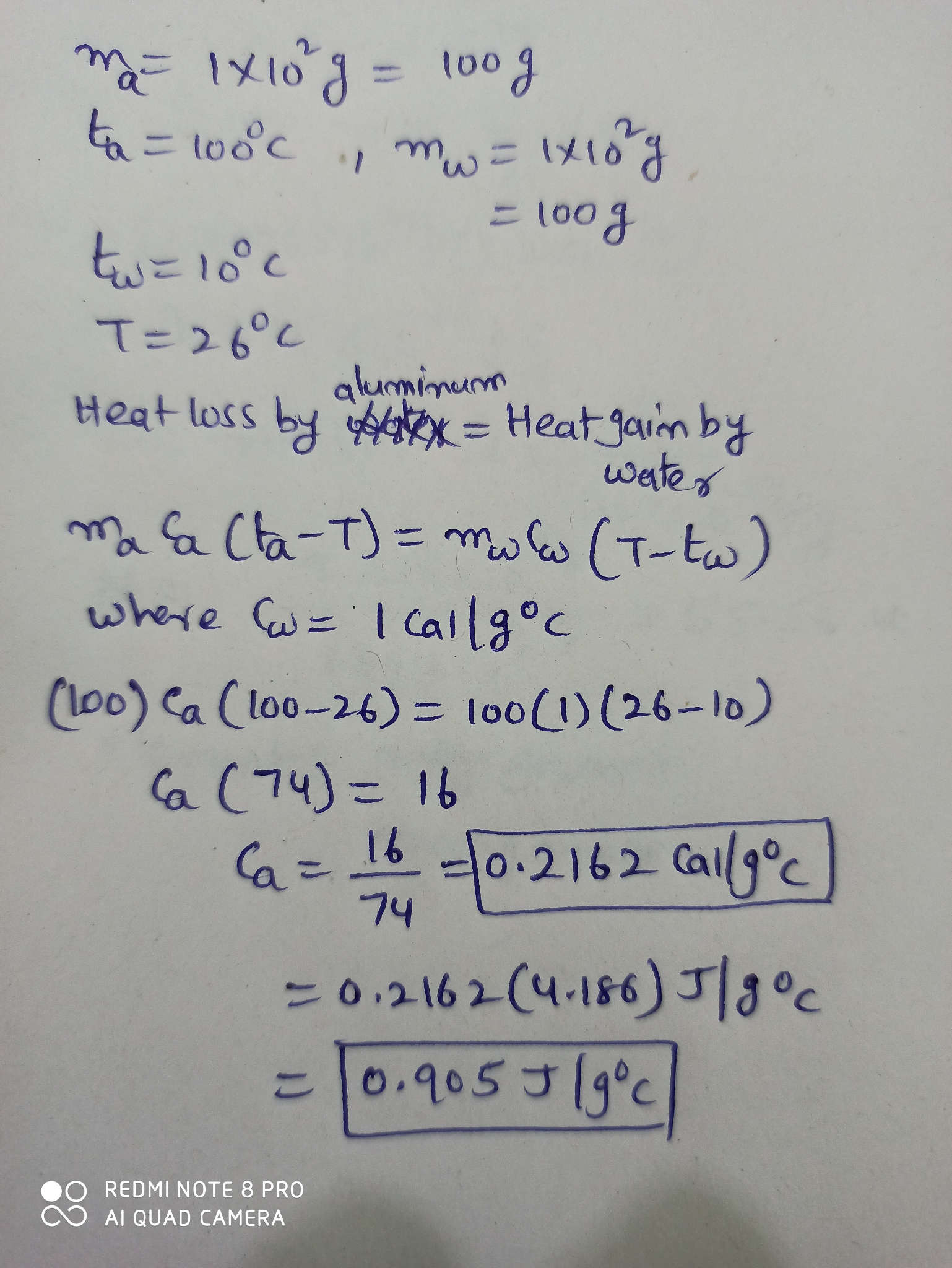4. specific heat of aluminium is 0.2162 cal/g ºC

5. specific heat of metal fishing weight is 0.1818 cal/g ºC

#### Earn Coin

Coins can be redeemed for fabulous gifts.

Similar Homework Help Questions
• ### A piece of metal of mass 35.0 g at 100.0°C was placed in 150.0 g of...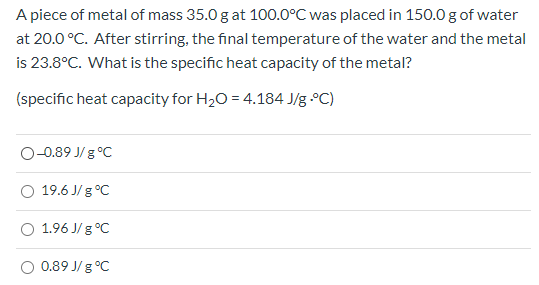A piece of metal of mass 35.0 g at 100.0°C was placed in 150.0 g of water at 20.0 °C. After stirring, the final temperature of the water and the metal is 23.8°C. What is the specific heat capacity of the metal? (specific heat capacity for H2O = 4.184 J/g °C) O-0.89 J 8°C 19.6 J/g °C 1.96J/g °C O 0.89 J/g °C

• ### A 275-g sample of nickel at 100.0°C is placed in 100.0 g of water at 22.0°C....

A 275-g sample of nickel at 100.0°C is placed in 100.0 g of water at 22.0°C. What is the final temperature of the water? Assume no heat transfer with the surroundings. The specific heat of nickel is 0.444 J/g·°C and the specific heat of water is 4.184 J/g·°C. Hint: The final temp for both the system and surroundings will be the same.

• ### A 41.0 g block of an unknown metal is heated in a hot water bath to...A 41.0 g block of an unknown metal is heated in a hot water bath to 100.0°C. When the block is placed in an insulated vessel containing 130.0 g of water at 25.0°C, the final temperature is 28.0°C. Determine the specific heat of the unknown metal. The cs for water is 4.18 J/g°C.    ____ J/g°C

• ### . A 150.0 g sample of a Metal was heated to 95.0°C. When the hot metal...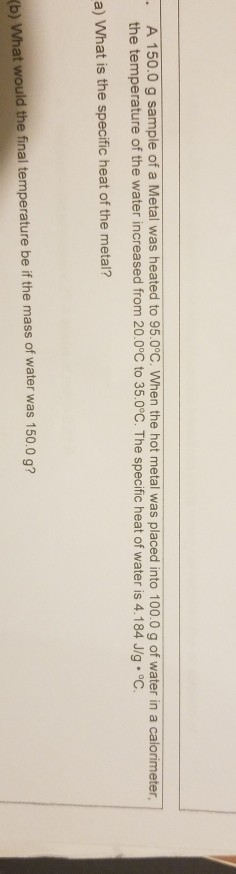. A 150.0 g sample of a Metal was heated to 95.0°C. When the hot metal was placed into 100.0 g of water in a calorimeter, the temperature of the water increased from 20.0°C to 35.0°C. The specific heat of water is 4.184 J/g °C. a) What is the specific heat of the metal? Kb) What would the final temperature be if the mass of water was 150.0 q?

• ### A 3.00-g sample of aluminum pellets (specific heat capacity=0.89 J/°C g) and a 18.50-g sample of...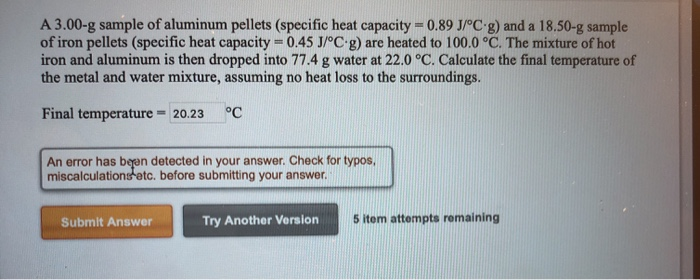A 3.00-g sample of aluminum pellets (specific heat capacity=0.89 J/°C g) and a 18.50-g sample of iron pellets (specific heat capacity = 0.45 J/°C-g) are heated to 100.0 °C. The mixture of hot iron and aluminum is then dropped into 77.4 g water at 22.0 °C. Calculate the final temperature of the metal and water mixture, assuming no heat loss to the surroundings. Final temperature = 20.23 °C An error has been detected in your answer. Check for typos. miscalculations...

• ### 3. A 2.00 g block of a metal (specific heat = 2.09 J g: Kl) at 80.0 °C is placed into container with 10.0 g liquid wate...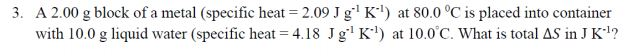3. A 2.00 g block of a metal (specific heat = 2.09 J g: Kl) at 80.0 °C is placed into container with 10.0 g liquid water (specific heat = 4.18 Jg' K ) at 10.0°C. What is total AS in JK?

• ### A 750 g block of copper is heated to 250 degrees C. It is placed in...

A 750 g block of copper is heated to 250 degrees C. It is placed in a 350 g aluminum vessel that contains 200 g of water at 5.00 degrees C. What is the final temperature of the copper, water, and aluminum vessel? Specific heat of copper = 0.093 cal/g C degrees Specific heat of aluminum = 0.215 cal/g C degrees Specific heat for water = 1.0 cal/g C degrees

• ### A 100. g sample of water at 25.3 C was placed in a calorimeter. 45.0 g...

A 100. g sample of water at 25.3 C was placed in a calorimeter. 45.0 g of lead shots (at 100 C) was added to the calorimeter and the final temperature of the mixture was 34.4 C. What is the specific heat of lead? 100.0g

• ### a 100.0 gram metal sample is heated to a temperature of 110 degrees celcius then placed into a calorimeter containing 100.0 r of pure water (at initial temperature of 23 degrees celcius)

a 100.0 gram metal sample is heated to a temperature of 110 degrees celcius then placed into a calorimeter containing 100.0 r of pure water (at initial temperature of 23 degrees celcius). after the sample and water reach thermal equalibrium, their final temperature is 29 degrees celsius. what is the specific heat capacity of the metal sample in calories/g*degrees celsius.

• ### An aluminum calorimeter with a mass of 425.00 g contains 1062.50 g of water. The calorimeter...

An aluminum calorimeter with a mass of 425.00 g contains 1062.50 g of water. The calorimeter and water are in thermal equilibrium at 12.50 ° C. Two metal blocks are placed in the water. One is a piece of copper from 212.50 g to 85.00 ° C. The other has a mass of 202.50 g and is originally at a temperature of 105.00 ° C. The entire system is stabilized at a final temperature of 22.50 ° C. (a) Determine...